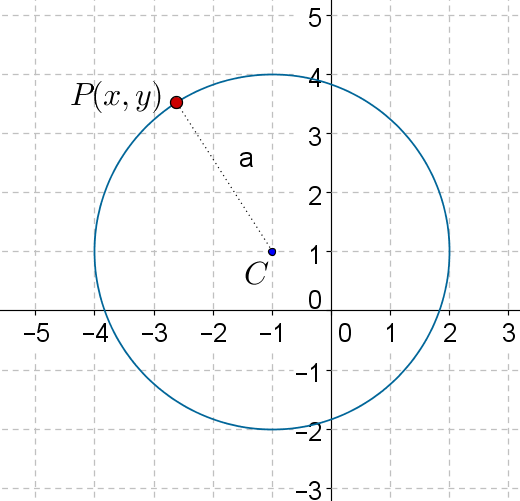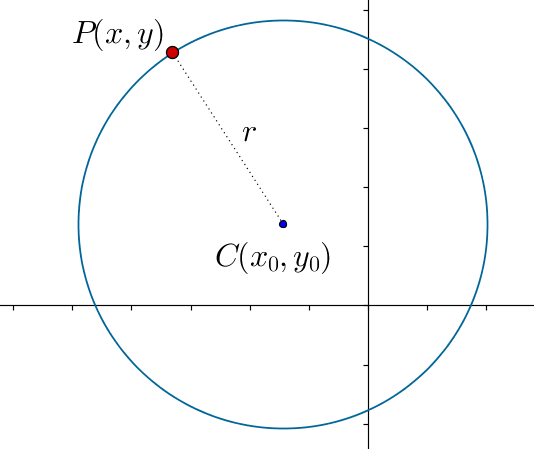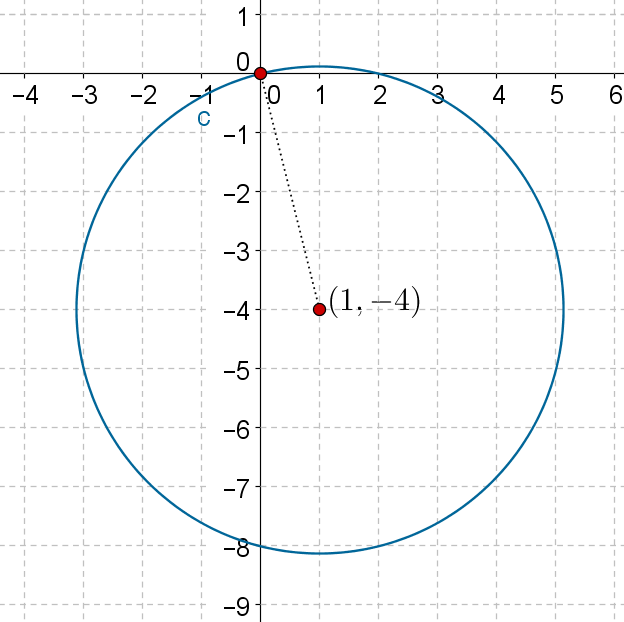# Circles With Arbitrary Centers

Circles With Arbitrary Centers

Consider the following figure, which shows a circle of radius 3 units centered at the point $$C\left( { - 1,1} \right)$$:What will be the equation of this circle?

Any point $$P\left( {x,y} \right)$$ lying on this circle will be at a distance of 3 units from the center $$C\left( { - 1,1} \right)$$ . Using the distance formula, we have:

\begin{align}&PC = 3\\&\Rightarrow \,\,\,\sqrt {{{\left( {x - \left( { - 1} \right)} \right)}^2} + {{\left( {y - 1} \right)}^2}} = 3\\&\Rightarrow \,\,\,\sqrt {{{\left( {x + 1} \right)}^2} + {{\left( {y - 1} \right)}^2}} = 3\\&\Rightarrow \,\,\,{\left( {x + 1} \right)^2} + {\left( {y - 1} \right)^2} = 9\end{align}

This is the equation which the coordinates of any point on the circle will satisfy (and no point not lying on the circle will satisfy) – hence, this is the equation of the circle.

In general, consider a circle with center $$C\left( {{x_0},{y_0}} \right)$$ and radius r:Any point $$P\left( {x,y} \right)$$ lying on this circle will be at a distance of r units from $$C\left( {{x_0},{y_0}} \right)$$ . Thus,

\begin{align}&PC = r\\&\Rightarrow \,\,\,\sqrt {{{\left( {x - {x_0}} \right)}^2} + {{\left( {y - {y_0}} \right)}^2}} = r\\&\Rightarrow \,\,\,{\left( {x - {x_0}} \right)^2} + {\left( {y - {y_0}} \right)^2} = {r^2}\end{align}

This is the required equation.

Example 1: Write the equation of the circle centered at the point $$\left( { - 2, - 3} \right)$$ and having a radius of 4 units.

Solution: The required equation will be

$\begin{array}{l}{\left( {x - \left( { - 2} \right)} \right)^2} + {\left( {y - \left( { - 3} \right)} \right)^2} = {4^2}\\ \Rightarrow \,\,\,{\left( {x + 2} \right)^2} + {\left( {y + 3} \right)^2} = 16\end{array}$

Example 2: Write the equation of the circle centered at $$\left( {1, - 4} \right)$$ and passing through the origin.

Solution: The following figure shows the specified circle:We can determine the radius r of this circle by calculating the distance between its center and the origin. We have:

$\begin{array}{l}{r^2} = {\left( {1 - 0} \right)^2} + {\left( { - 4 - 0} \right)^2} = 17\\ \Rightarrow \,\,\,r = \sqrt {17} \end{array}$

Thus, the equation of this circle will be

$\begin{array}{l}{\left( {x - 1} \right)^2} + {\left( {y - \left( { - 4} \right)} \right)^2} = {\left( {\sqrt {17} } \right)^2}\\ \Rightarrow \,\,\,{\left( {x - 1} \right)^2} + {\left( {y + 4} \right)^2} = 17\end{array}$

Download Coordinate Geometry Worksheets
Coordinate Geometry
Grade 9 | Questions Set 1
Coordinate Geometry
Grade 9 | Answers Set 1
Coordinate Geometry
Grade 10 | Questions Set 1
Coordinate Geometry
Grade 10 | Answers Set 1
More Important Topics
Numbers
Algebra
Geometry
Measurement
Money
Data
Trigonometry
Calculus